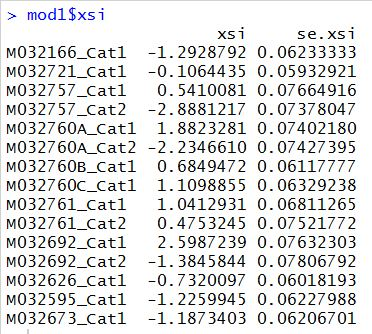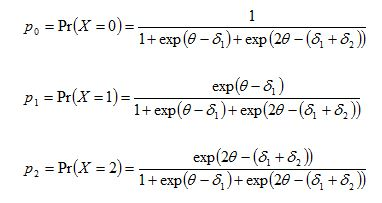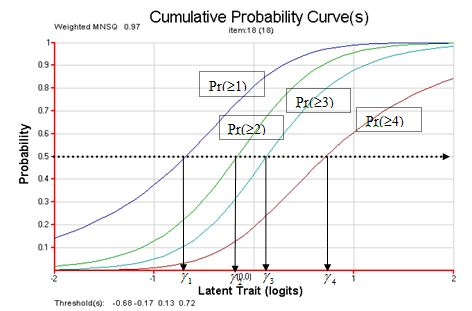## Tutorial 5:  Partial Credit Model(2013-7-31)

Home
1. Install R
2. Install TAM
3. Rasch Model
4. CTT, Fit
5. Partial Credit Model
6. Population Model
7. DIF

More resources

## Summary of Tutorial

This tutorial shows how to recode raw and missing item responses, run IRT partial credit model and obtain Masters' delta parameters and Thurstonian thresholds. The tutorial also shows how to anchor item parameters while computing ability estimates. For an extension, the tutorial shows how score equivalence tables can be produced, illustrating that test scores are sufficient statistics for ability estimates.

## Data files

The data file is from TIMSS 2011 mathematics tests, released items, blocks 1 and 14, for Taiwan and Australian students. The test paper and the data file can be downloaded through the following links:

TIMSS released items block 1
TIMSS 2011 Taiwan and Australian data in csv format

TIMSS data file contains double-digit codes for constructed response items and raw responses for multiple-choice items. To run an IRT analysis using TAM, the data need to be recoded and scored first. The following is an excerpt of the data file.## R script file

Before TAM functions can be called, the TAM library has to be loaded into the R workspace. Line 2 loads the TAM library.

Line 5 sets the working directory and line 6 reads the data file "TIMSS_AUS_TWN_rawresp.csv" into a variable called "raw_resp".
setwd("C:/G_MWU/TAM/Tutorials/data")

### Recode data file

Line 9 extracts the first 11 columns of the data file, as columns 12 - 14 contain country code, gender code and book ID respectively.
resp <- raw_resp[,1:11]

When responses are missing in the csv file (i.e.,"blanks"), R read them in as "NA" (Not Available). NA is a reserved word in R for missing data. Line 10 finds records with all missing item responses.  (e.g, record 192 in raw_resp).
all.na <- apply(resp, 1, function(x){all(is.na(x))})

Line 11 removes records in which all responses are missing (NA)
resp <- resp[!all.na,]

If you intend to delete records containing any NA (i.e., some NA and some valid responses in a record), then the function "na.omit" can be used. na.omit does listwise deletion.

Line 12 recodes double-digit codes 20 and 21 to 2.
resp[resp==20 | resp==21] <- 2

Similar recodes are performed in lines 13 and 14.

### Differentiate between "omit" and "not-reached"

TIMSS data provide different codes for "omit" and "not-reached" missing responses. "omit" refers to missing responses embedded in a response string, while "not-reached" refers to missing responses at the end of a response string. There are no fixed rules for the treatment of "omit" and "not-reached" responses, but one convention is that when item parameters are estimated, "not-reached" responses are treated as missing while "omit" responses are treated as incorrect. For abililty estimates, all missing responses (omit or not-reached) are treated as incorrect. In this example, we will use this convention.

Line 15 recodes "99" (omit) to zero (incorect). Line 16 recodes "96" and "6" (not-reached) to NA (missing).
resp[resp==99] <- 0
resp[resp==96 | resp==6] <- NA

### Score multiple-choice items

Double-digit codes for constructed response items have been recoded in lines 12-16, but multiple-choice items still have raw responses. Before the tam function can be called, the MC items need to be scored. In Tutorial 4, we used a key statement to score responses. In this tutorial, we will score groups of items with the same key (e.g., key is B).
The key for item 9 is D (or 4). The key for items 1 and 2 is B (or 2). The key for items 10 and 11 is C (or 3).
Scored <- resp
Scored[,9] <- (resp[,9]==4)*1
Scored[,c(1,2)] <- (resp[,c(1,2)]==2)*1
Scored[,c(10,11)] <- (resp[,c(10,11)]==3)*1

### Run IRT analysis (MML)

Line 25 runs an IRT analysis using MML estimation. The results of the IRT analysis are stored in variable "mod1".
mod1 <- tam(Scored)
The tam function automatically detects that some item responses are partial credit (maximum score more than 1), and tam will fit a Rasch partial credit model by default. There is no need to specify a partial credit model. However, for more complex models (e.g., generalised partial credit model, facets models), the IRT model can be explicitly specified.

### Item parameters of the partial credit model

Line 28 asks for a display of item parameters estimated.
mod1\$xsi
The estimated item parameters are shown below:These item parameters are sometimes known as the "delta" parameters, as in Masters' derivation of the partial credit model (Masters, 1982). For example, for a partial credit item with 0, 1, 2 scores, the probabilities of being in the three response categories areThe delta parameters are also sometimes known as uncentralised thresholds  e.g., in the RUMM software (RUMM Laboratory, 1998). The delta parameters are the intersection points of adjacent category probability curves. They are useful for the mathematical formualation of the partial credit model. They are not useful for interpreting item difficulties of the response categories. Thurstonian thresholds are more helpful as representing category difficulties, even though the whole notion of item difficulty in the context of the partial credit model needs to be more widely discussed.

### Thurstonian thresholds

The Thurstonian threshold for a score category is defined as the ability at which the probability of achieving that score or higher reaches 0.50. TheThurstonian thresholds are shown graphically below.Line 31 runs the Thurstonian threshold function
tthresh <- tam.threshold(mod1)

The following shows the Thurstonian thresholds:Lines 35-37 make a plot of the Thurstonian thresholds in a new window, as shown below:
windows (width=8, height=7)
par(ps=9)
dotchart(t(tthresh), pch=19)### Plot expected scores curve

Line 41 plots the expected scores curve.

### Re-run analysis with "not-reached" responses scored as incorrect

To obtain ability estimates, the "not-reached" responses will be treated as incorrect. However, the item difficulty parameters will be anchored at the estimates obtained from the previous run.

Lines 44 and 45 re-score the responses so that missing responses (NA) are now scored as 0.
Scored2 <- Scored
Scored2[is.na(Scored2)] <- 0

To prepare for anchoring item parameters, set up a matrix where the first column is a sequence of parameter numbers, and the second column contains the anchoring item parameters. Lines 48-50 set up the anchoring matrix.
nparam <- length(mod1\$xsi\$xsi)
xsi <- mod1\$xsi\$xsi
anchor <- matrix(c(seq(1,nparam),xsi), ncol=2)

Line 53 re-runs the IRT model with item anchors. The results are stored in variable "mod2".
mod2 <- tam(Scored2, xsi.fixed=anchor)

Ability estimates are obtained in line 56, based on the results in "mod2".
ability <- tam.wle(mod2)

## Extension

### Score Equivalence Table

Under the Rasch model, respondents' test scores are sufficient statistics for their ability estimates. This means that irrespective of which items a respondent answered correctly, the total score on the test determines a respondent's ability. For this reason, it is possible to obtain a score equivalence table showing a table of test scores and corresponding IRT abilities. An easy way to do this is to create a set of dummy item responses with all possible test scores, run an IRT analysis with item parameters anchored at the values obtained from the larger data set. Lines 72-75 of the R script file create such a set of dummy item responses.
dummy <- matrix(0,nrow=16,ncol=11)
dummy[lower.tri(dummy)] <- 1
dummy[12:16,c(3,4,7,8)][lower.tri(dummy[12:16,c(3,4,7,8)])]<-2
colnames(dummy) <- paste("I",seq(1:11),sep="")
The dummy data set is shown below:Run an IRT analysis with the dummy data set and anchor the item parameters using the variable "anchor" that was set up earlier.
mod3 <- tam(dummy, xsi.fixed=anchor)
wle3 <- tam.wle(mod3)
The ability estimates from this run are shown below.The score equivalence table can be used to assign ability estimates corresponding to test scores. So if the test is used in the future, we do not need to run an IRT analysis. We can just use the score equivalence table to find out IRT abilities, unless, of course, there are reasons to believe that the item difficulties have changed.

As an exercise, change the item responses in the variable "dummy", so that correct responses are to a different set of questions. For example, use the following code to generate item responses
dummy2 <- matrix(0,nrow=16,ncol=11)
dummy2[upper.tri(dummy)] <- 1
dummy2[12:16,] <-1
dummy2[12:16,c(2,3,4,7,8)][upper.tri(dummy[12:16,c(2,3,4,7,8)])]<-2
colnames(dummy2) <- paste("I",seq(1:11),sep="")
Re-run the IRT analysis using anchoring.
How does the score equivalence table compare with the one obtained previously?
How does the WLE reliability (WLE.rel) compare with the previous one?
Will there be any difference between item fit statistics using the original (real) data set and the dummy data sets?

## References

Masters, G. N. (1982) A Rasch Model for partial credit scoring. Psychometrika, 47, 149-174.